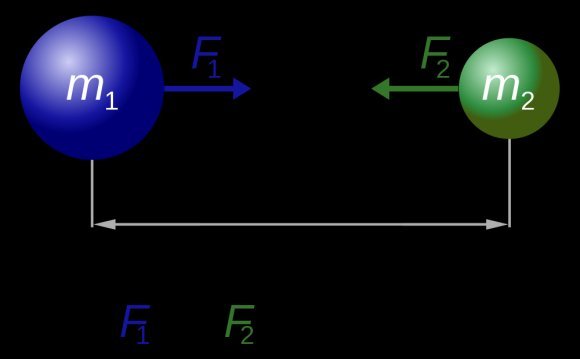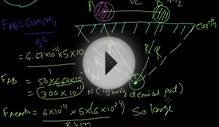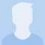# Gravitational force between two objects

April 24, 2015Weight is the force generated by the gravitational attraction on the rocket. We are more familiar with weight than with the other forces acting on a rocket, because each of us have our own weight which we can measure every morning on the bathroom scale. We know when one thing is heavy and when another thing is light. But weight, the gravitational force, is fundamentally different from the other forces acting on a rocket in flight. The aerodynamic forces, lift and drag, and the thrust force are mechanical forces. The rocket must be in physical contact with the gases which generate these forces. The gravitational force is a field force and the rocket does not have to be in contact with the source of this force.

The nature of the gravitational force has been studied by scientists for many years and is still being investigated by theoretical physicists. For an object the size of a rocket, the explanation given three hundred years ago by Sir Isaac Newton is sufficient to describe the motion of the object. Newton developed his theory of gravitation when he was only 23 years old and published the theories with his laws of motion some years later. As Newton observed, the gravitational force between two objects depends on the mass of the objects and the inverse of the the square of the distance between the objects. More massive objects create greater forces and the farther apart the objects are the weaker the attraction. Newton was able to express the relationship in a single weight equation. The gravitational force, , between two particles equals a universal constant, G, times the product of the mass of the particles, m1 and m2, divided by the square of the distance, d, between the particles.

F = G * m1 * m2 / d^2

If you have a lot of particles acting on a single particle, you have to add up the contribution of all the individual particles. For objects near the Earth, the sum of the mass of all the particles is simply the mass of the Earth and the distance is then measured from the center of the Earth. On the surface of the Earth the distance is about 4000 miles. Scientists have combined the universal gravitational constant, the mass of the Earth, and the square of the radius of the Earth to form the Earth's gravitational acceleration, ge .

Source: www.grc.nasa.gov
##### INTERESTING VIDEOGravitational Force between Two SpheresGravitational ForceWhy Gravitational Force between two objects on Earth is ...

##### INTERESTING FACTS##### What increases gravitational force between two objects?

When two objects get closer to each other increases the gravitational force between them increases.